﻿

### Weak$^*$ closures and derived sets in dual Banach spaces

#### Abstract

The main results of the paper: \textbf{(1)} The dual Banach space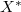$X^*$ contains a linear subspace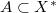$A\subset X^*$ such that the set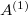$A^{(1)}$ of all limits of weak$^*$ convergent bounded nets in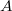$A$ is a proper norm-dense subset of$X^*$ if and only if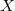$X$ is a non-quasi-reflexive Banach space containing an infinite-dimensional subspace with separable dual. \textbf{(2)} Let$X$ be a non-reflexive Banach space. Then there exists a convex subset$A\subset X^*$ such that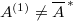$A^{(1)}\neq {\overline{A}\,}^*$ (the latter denotes the weak$^*$ closure of$A$). \textbf{(3)} Let$X$ be a quasi-reflexive Banach space and$A\subset X^*$ be an absolutely convex subset. Then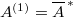$A^{(1)}={\overline{A}\,}^*$.

DOI Code: 10.1285/i15900932v31n1p129

Keywords: norming subspace ; quasi-reflexive Banach space ; total subspace ; weak$^*$ closure ; weak$^*$ derived set ; weak$^*$ sequential closure

Full Text: PDF